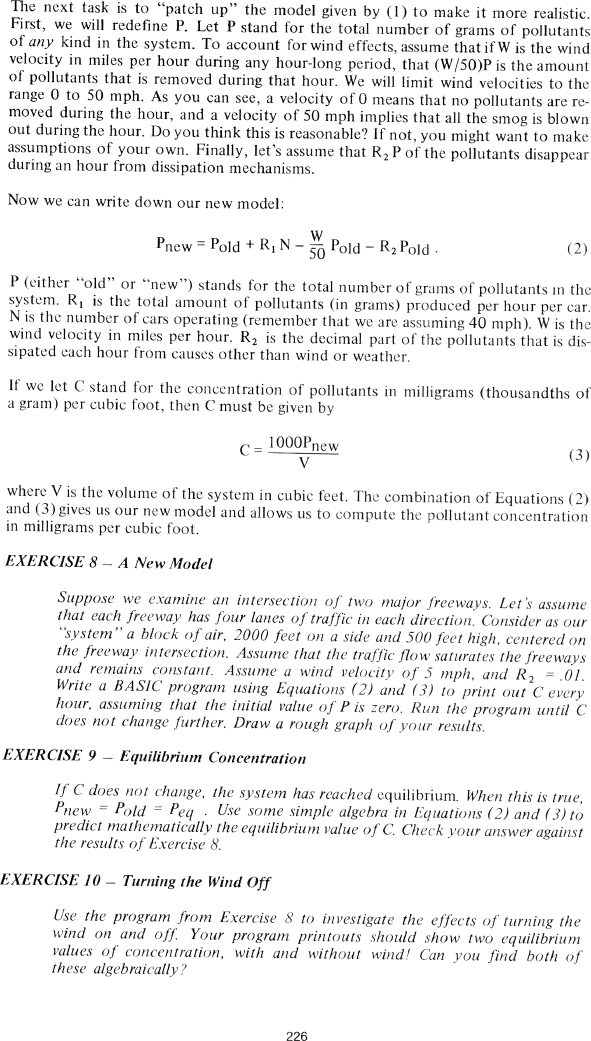The Best of Creative Computing Volume 1 (published 1976)

 `Page 226 << PREVIOUS >> NEXT Jump to page: Go to contents Go to thumbnails`

The Automobile and Air Pollution (new model, equilibrium concentration, turning the wind off)```The next task is to "patch up" the model given by (1) to make it more realistic.
First, we will redefine P. Let P stand for the total number of grams of
pollutants of any kind in the system. To account for wind effects, assume that
if W is the wind velocity in miles per hour during any hour-long period, that
(W/50)P is the amount of pollutants that is removed during that hour. We will
limit wind velocities to the range 0 to 50 mph. As you can see, a velocity of 0
means that no pollutants are removed during the hour, and a velocity of 50 mph
implies that all the smog is blown out during the hour. Do you think this is
reasonable? If not, you might want to make assumptions of your own. Finally,
let's assume that R2 P of the pollutants disappear during an hour from
dissipation mechanisms.

Now we can write down our new model:

Pnew=Pold+R1N-W/50Pold-R2Pold. (2)

P (either "old" or "new") stands for the total number of grams of pollutants in
the system. R1 is the total amount of pollutants (in grams) produced per hour
per car. N is the number of cars operating (remember that we are assuming 40
mph). W is the wind velocity in miles per hour. R2 is the decimal part of the
pollutants that is dissipated each hour from causes other than wind or weather.

If we let C stand for the concentration of pollutants in milligrams (thousandths
of a gram) per cubic foot, then C must be given by

C = 1000Pnew/V	(3)

where V is the volume of the system in cubic feet. The combination of Equations
(2) and (3) gives us our new model and allows us to compute the pollutant
concentration in milligrams per cubic foot.

EXERCISE 8 - A New Model

Suppose we examine an intersection of two major freeways. Let is assume that
each freeway has four lanes of traffic in each direction, Consider as our
'system " a block of air, 2000 feet on a side and 500 feet high, centered on the
freeway intersection. Assume that the traffic flow saturates the freeways and
remains constant. Assume a wind velocity of 5 mph, and R2 = .01. Write a BASIC
program using Equations (2) and (3) to print out C every hour, assuming that the
initial value of P is zero. Run the program until C does not change further.
Draw a rough graph of your results.

EXERCISE 9 - Equilibrium Concentration

If C does not change, the system has reached equilibrium. When this is true,
Pnew = Pold = Peq . Use some simple algebra in Equations (2) and (3) to predict
mathematically the equilibrium value of C. Check your answer against the results
of Exercise 8.

EXERCISE 10 - Turning the Wind Off

Use the program from Exercise 8 to investigate the effects of turning the wind
on and off. Your program printouts should show two equilibrium values of
concentration, with and without wind! Can you find both of these algebraically?

226Page 226        << PREVIOUS        >> NEXT        Jump to page:                Go to contents        Go to thumbnails

<!--